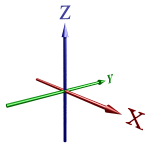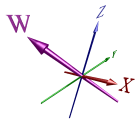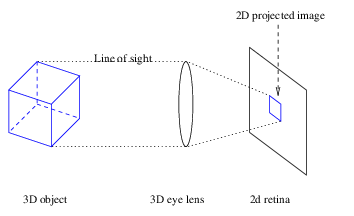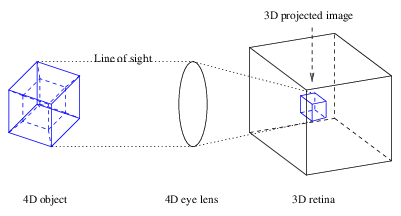# 4D Visualization

## Introduction

### Higher-Dimensional Space

The world around us exists in 3-dimensional (3D) space. There are 3 pairs of cardinal directions: left and right, forward and backward, and up and down. All other directions are simply combinations of these fundamental directions. Mathematically, these pairs of directions correspond with three coordinate axes, which are conventionally labelled X, Y, and Z, respectively.The arrows in the diagram indicate which directions are considered numerically positive and which are negative. By convention, right is positive X, left is negative X, forward is positive Y, backward is negative Y, and up is positive Z, and down is negative Z. We shall refer to these directions as +X, -X, +Y, -Y, +Z, and -Z, respectively. The point where the coordinate axes intersect is called the origin.

As far as we know, the space we inhabit consists of these 3 dimensions, and no more. We may think that space has to be 3-dimensional, that it can't possibly be anything else. Physically, this may be true, but mathematically, there is nothing special about the number 3 that makes it the only possible number of dimensions space can have. It is possible to have dimensions lower than 3: for example, 1D space consists of a single straight line stretching off to infinity at either end; and 2D space consists of a flat plane, extending in length and width indefinitely. However, nothing about geometry restricts us to 3 dimensions or less. It is quite possible—and mathematically straightforward—to deal with geometry in more than 3 spatial dimensions. In particular, we can have a 4th spatial dimension that lies perpendicular to all 3 of the familiar cardinal directions in our world. The space described by these 4 dimensions is called 4-dimensional space, or 4D space for short.

In a 4D world, there is another directional axis which is perpendicular to the X, Y, and Z axes. We shall label this axis W, and call the direction along this axis the fourth direction. This new axis also has positive and negative directions, which we shall refer to as +W and -W.It is important to understand that the W-axis as depicted here is perpendicular to all of the other coordinate axes. We may be tempted to try to point in the direction of W, but this is impossible because we are confined to 3-dimensional space.

### Why Bother?

Why bother trying to visualize a higher-dimensional space that we can neither experience nor access directly? Besides pure curiosity, 4D visualization has a wide variety of useful applications.

Mathematicians have long wondered how to visualize 4D space. In calculus, a very useful method of understanding functions is to graph them. We can plot a real-valued function of one variable on a piece of graph paper, which is 2D. We can also plot a real-valued function of two variables using a 3D graph. However, we run into trouble with even the simplest complex-valued function of 1 complex argument: every complex number has two parts, the real part and the imaginary part, and requires 2 dimensions to be fully depicted. This means that we need 4 dimensions to plot the graph of the complex function. But to see the resulting graph, one must be able to visualize 4D.

Einstein's theory of Special Relativity postulates that space and time are interrelated, forming a space-time continuum of 3 spatial dimensions and 1 temporal dimension. While it is possible to visualize space-time simply by treating time as time and examining “snapshots” of space-time objects at various points in time, it is also useful to treat space-time geometrically. For example, the distance between two events is the distance between two 4D points. The light-cone also has a particular shape that can only be adequately visualized as a 4D object.

Furthermore, Einstein's theory of General Relativity describes curvature in space-time. While it may not actually be a curvature into a physical spatial dimension, it is helpful to visualize it as such, so that we can see how space curves in 4D as a 3-manifold. If space in the universe had positive curvature, for example, it would be in the shape of a 4D hypersphere—but what exactly does that look like?

Many other interesting mathematical objects also require 4D visualization to be appreciated fully. Among them are 4D polytopes (4D equivalents of polyhedra), topological objects such as the 3-torus and the Real Projective Plane which can only be embedded without self-intersection in 4D or higher, and the quaternions, which are useful for representing 3D rotations. It is difficult to fully appreciate these objects without being able to see them in their native space.

### Is it possible to visualize 4D?

Some believe that it is impossible for us to visualize 4D, since we are confined to 3D and therefore cannot directly experience it. However, it is possible to develop a good idea of what 4D objects look like: the key lies in the fact that to see N dimensions, one only needs an (N-1)-dimensional retina.

Even though we are 3D beings who live in a 3D world, our eyes actually only see in 2D. Our retina has only a 2D surface area with which it can detect light coming into our eye. What our eye sees is in fact not 3D, but a 2D projection of the 3D world we are looking at.In spite of this, we are quite able to grasp the concept of 3D. Our mind is quite facile at reconstructing a 3D model of the world around us from the 2D images seen by our retina. It does this by using indirect information in the 2D images such as light and shade, parallax, and previous experience. Even though our retina doesn't actually see 3D depth, we instinctively infer it. We have a very good intuitive grasp of what 3D is, to the point that we are normally quite unconscious of the fact we're only seeing in 2D.

Similarly, a hypothetical 4D being would have a 3D retina, and would see the 4D world as 3D projections.It would not directly see the 4th dimension, but would infer it using indirect information such as light and shade, parallax, and previous experience.

The key here is that what the 4D being sees in its retina is 3-dimensional, not 4-dimensional. The 4th dimension is inferred. But since we have a good intuitive grasp of 3D, it is not that difficult to understand what a 4D being sees in its retina. From there, we just need to learn how to infer 4D depth.

The rest of this document will describe in detail the basic principles of 4D visualization, as well as provide a number of examples of 4D objects. We shall take a purely geometrical approach and treat all 4 dimensions as spatial dimensions.

Last updated 06 Feb 2018.